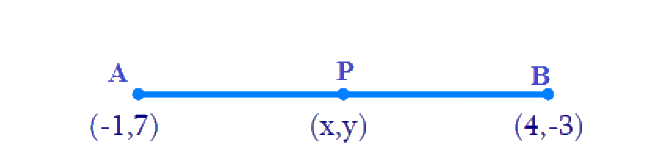# Ex.7.2 Q1 Coordinate Geometry Solution - NCERT Maths Class 10

Go back to  'Ex.7.2'

## Question

Find the coordinates of the point which divides the join of $$(-1, 7)$$ and $$(4, -3)$$ in the ratio $$2:3$$.

Video Solution
Coordinate Geometry
Ex 7.2 | Question 1

## Text Solution

Reasoning:

The coordinates of the point $$P(x, y)$$ which divides the line segment joining the points $$A(x_1, y_1)$$ and $$B(x_2, y_2)$$, internally, in the ratio $$m_1 : m_2$$ is given by the Section Formula

\begin{align}\!P(x,y) \!=\! \left[ {\frac{{mx_2} \!+\! {nx_1}}{{m \!+ n}},\frac{{my_2} \!+\! {ny_1}}{{m} +\! {n}}}\! \right]\! \end{align}

What is Known?

The $$x$$ and $$y$$ co-ordinates of the points which is to be divided in the ratio $$2:3$$

What is Unknown?

The coordinates of the point which divides the join of $$(-1, 7)$$ and $$(4, -3)$$ in the ratio $$2:3$$

Steps:

Given,

• Let $$P(x, y)$$ be the required point.• Let $$A(-1, 7)$$ and $$B(4, -3)$$
• $$m: n = 2:3$$
• Hence
• $$x_1 = -1$$
• $$y_1 = 7$$
• $$x_2 = 4$$
• $$y_2 = -3$$

By Section formula

\begin{align}\!P(x,y) \!=\! \left[ {\frac{{mx_2} \!+\! {nx_1}}{{m \!+ n}},\frac{{my_2} \!+\! {ny_1}}{{m} +\! {n}}}\! \right]\!\;\;\dots(1) \end{align}

By substituting the values in the Equation (1)

\begin{align}x &= \frac{{2 \times 4 + 3x( - 1)}}{{2 + 3}} \\x &= \frac{{8 - 3}}{5}\\x &= \frac{5}{5} = 1\\\\ y &= \frac{{2 \times ( - 3) + 3 \times 7}}{{2 + 3}} \\y &= \frac{{ - 6 + 21}}{5} \\y &= \frac{{15}}{5} = 3\end{align}

Therefore, the co-ordinates of point $$P$$ are $$(1, 3)$$.

Learn from the best math teachers and top your exams

• Live one on one classroom and doubt clearing
• Practice worksheets in and after class for conceptual clarity
• Personalized curriculum to keep up with school